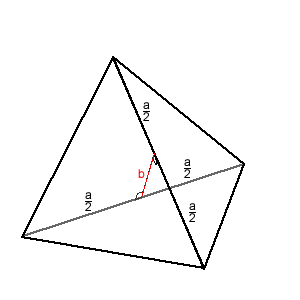#### You may also like### Just Rolling Round

P is a point on the circumference of a circle radius r which rolls, without slipping, inside a circle of radius 2r. What is the locus of P?### Coke Machine

The coke machine in college takes 50 pence pieces. It also takes a certain foreign coin of traditional design...### Just Opposite

A and C are the opposite vertices of a square ABCD, and have coordinates (a,b) and (c,d), respectively. What are the coordinates of the vertices B and D? What is the area of the square?

# Sliced

##### Age 14 to 16Challenge LevelAn irregular tetrahedron has two opposite sides the same length ($a$ say) and the line joining their midpoints is perpendicular to these two edges and is of length $b$. What is the volume of the tetrahedron?